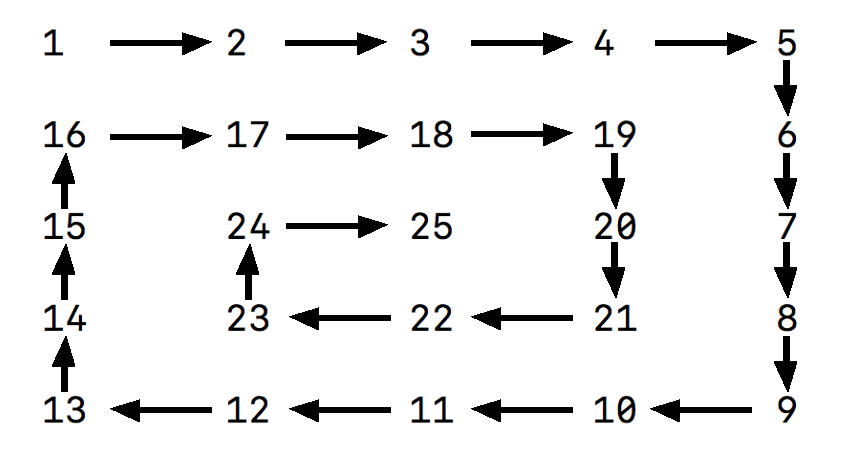MTH4300 Home

# MTH 4300: Midterm 2 Practice 6

Problem 1.

The user input consists of two positive integers $$R$$ and $$S$$. Create a program that calculates the total number of integers $$k$$ that satisfy $$R\leq k\leq S$$ and that are divisible by $$7$$ but not by $$5$$.

Problem 2.

When the product "ice cream" is sold, it has the base price and the tax applied to it. Different states have different taxes, but the base price stays the same. Create the class IceCream that has private attributes flavor, basePrice, and taxPercentRate; and appropriate public methods and constructors, including the following public methods: setFlavor, setBasePrice, setTaxPercentRate, getFlavor, and getTotalPrice. The class should also offer a constructor with two parameters: one of type IceCream and another of type double. This constructor will be used in the following way

IceCream B(A, newTax);


It should create an object called $$B$$ that has the same flavor and basePrice as $$A$$. However the taxPercentRate should be newTax instead of A.taxPercentRate. Provide a precise definition of the class and the implementation of the methods using the proper syntax of C++. When your class is applied to the following main function

int main(){
cout<<" dollars for ice cream with flavor=" <<iceCreamAlaska.getFlavor()<<endl;
cout<<"Customers in Texas have to pay "<<iceCreamTexas.getTotalPrice();
cout<<" dollars for ice cream with flavor=";
cout<<iceCreamTexas.getFlavor()<<endl;
}


the program should print the following

Customers in Alaska have to pay 33 dollars for ice cream with flavor=chocolate
Customers in Texas have to pay 36 dollars for ice cream with flavor=chocolate


Problem 3.

What happens when the following program is executed? Provide a rigorous justification for your answer.

 #include<iostream>
int main(){
int value1=0;
int value2=0;
int* p;
p=new int;
*p=14;
*(p+1)=3;
for(int i=2;i<100;++i){
p[i]=p[i-2];
}
for(int i=0;i<100;++i){
if( i+p[i] == 18){
value1+=p[i];
for(int j=0;j<100;++j){
value2+=p[j];
}
}
}
std::cout<<value1<<" "<<value2<<std::endl;
return 0;
}


Problem 4.

What is printed on standard output when the following program is executed? Provide a rigorous justification for your answer.

// mysteryPrinting.cpp
// compile with
// c++ mysteryPrinting.cpp -o mPrinting -std=c++11 -fno-elide-constructors
// execute with
// ./mPrinting
#include<iostream>
class Wolf{
private:
std::string name;
public:
Wolf(const std::string & = "");
Wolf(const Wolf &);
Wolf(Wolf &&);
void operator=(const Wolf &);
void operator=(Wolf &&);
virtual ~Wolf();
};
Wolf::Wolf(const std::string & s){
name=s;
}
Wolf::Wolf(const Wolf& x){
name="A"+x.name;
}
Wolf::Wolf(Wolf&& x){
name="B"+x.name;
x.name+="C";
}
void Wolf::operator=(const Wolf & x){
name+="D"+x.name;
}
void Wolf::operator=(Wolf && x){
name+="E"+x.name;
x.name+="F";
}
Wolf::~Wolf(){
std::cout<<name<<std::endl;
}
Wolf whisper(Wolf z){
Wolf w("G");
Wolf r=z;
return w;
}
int main(){
Wolf a("X");
Wolf b("Y");
b=whisper(a);
return 0;
}


Problem 5.

The user input consists of a positive integer $$n$$. Create a program that prints the $$n\times n$$ table that contains the numbers $$1$$, $$2$$, $$\dots$$, $$n^2$$ arranged in a spiral. An example for a spiral for $$n=5$$ is shown in the picture below.